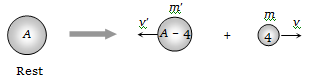# NEET Physics Nuclei Questions Solved

A radioactive nucleus undergoes $\mathrm{\alpha }$-emission to form a stable element. What will be the recoil velocity of the daughter nucleus if V is the velocity of $\mathrm{\alpha }$-emission and A is the atomic mass of radioactive nucleus
(a) $\frac{4\mathrm{V}}{\mathrm{A}-4}$             (b) $\frac{2\mathrm{V}}{\mathrm{A}-4}$
(c) $\frac{4\mathrm{V}}{\mathrm{A}+4}$             (d) $\frac{2\mathrm{V}}{\mathrm{A}+4}$

(a)According to conservation of momentum $4\mathrm{v}=\left(\mathrm{A}-4\right)\mathrm{v}\text{'}⇔\mathrm{v}\text{'}=\frac{4\mathrm{v}}{\mathrm{A}-4}$ .

Difficulty Level:

• 45%
• 28%
• 23%
• 6%